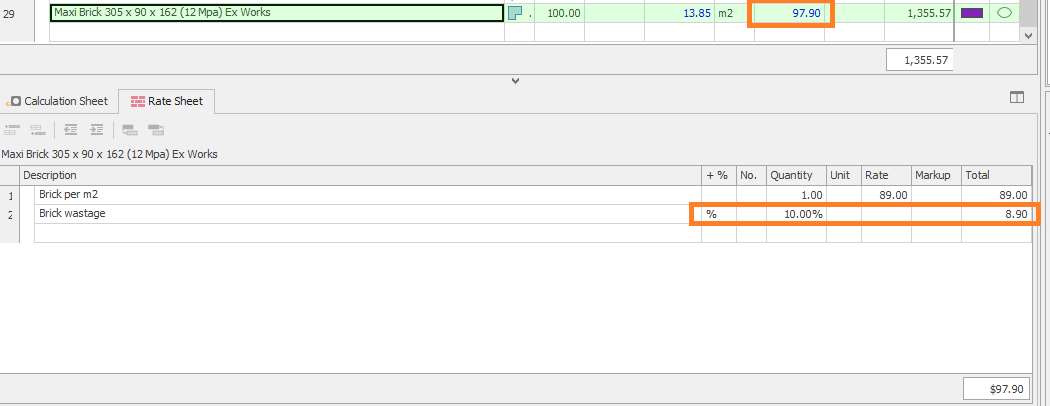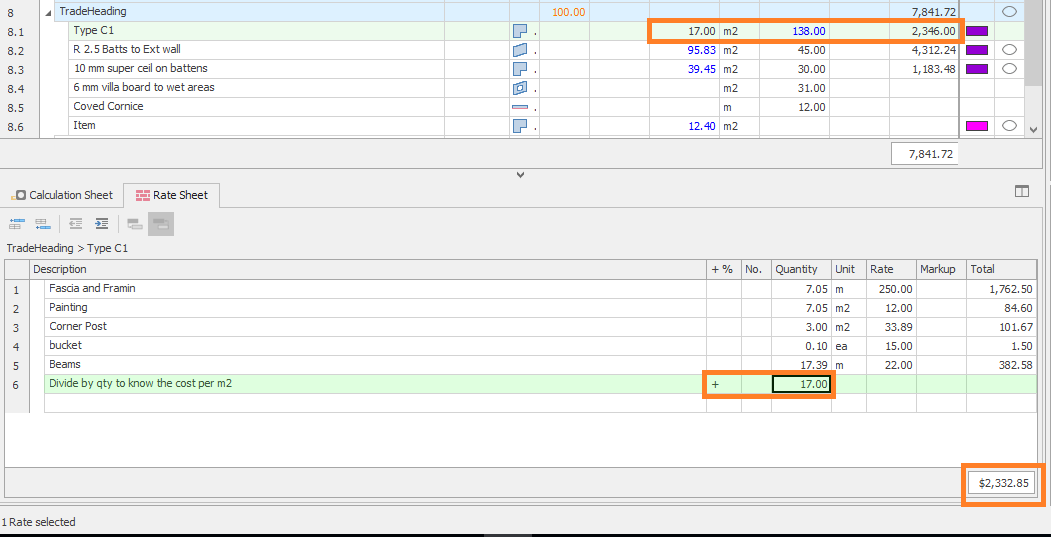# Usage of % and + Functions in Rate Sheet

Percentage Markup Rate sheet level:

Using the % function you can markup each items making up the composite rate.

When the % is used in the rate sheet, it calculates the percentage of the item total above its row and displays the value as a separate line item below. It is most commonly used to work out/displaying additional wastage cost to the rate sheet item.

This can also be used to give a discount to one item by typing a negative number. This field will be blank if no markup or discount is required for an item. The markup is applied before subsequent markups and adjustments in the Estimate sheet and for the overall Job.Fig1. Percentage Markup

Dividing Rate by Quantity/ Rate per square meter:

You can use the + Function to Calculate Total cost per Quantity specified, i.e. the total amount on the item will be divided by the qty of the Rate item where + function.

This is most commonly used to calculate the cost per m2. In the example below the Composite Rate is for the Total price of the Supply and install for an area of 17m2.Fig2. Composite Rate

To know the cost of the building the same area for per meter square, in the rate sheet add another item and use the + Function and set the quantity of the item. The final rate is the cost of building for 1m2.Fig3. Total cost per Quantity

0 out of 0 found this helpful